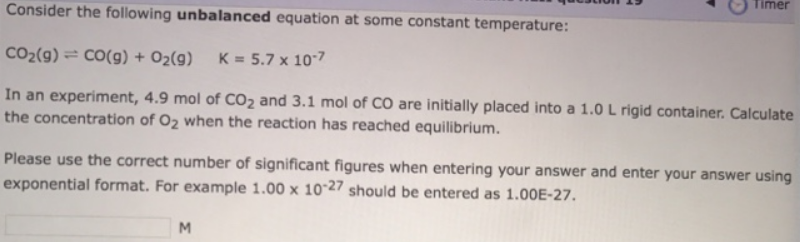# Problem: Consider the following  unbalanced equation at some constant temperature: CO2 (g) ⇌ CO (g) + O2 (g)       K = 5.7 x 10-7 In an experiment, 4.9 mol of CO2 and 3.1 mol of CO are initially placed into a 1.0 L rigid container. Calculate the concentration of O2 when the reaction has reached equilibrium. Please use the correct number of significant figures when entering your answer and enter your answer using exponential format. For example 1.00 x 10-27 should be entered as 1.00E-27.

###### FREE Expert Solution
88% (62 ratings)###### Problem Details

Consider the following  unbalanced equation at some constant temperature:

CO2 (g) ⇌ CO (g) + O2 (g)       K = 5.7 x 10-7

In an experiment, 4.9 mol of CO2 and 3.1 mol of CO are initially placed into a 1.0 L rigid container. Calculate the concentration of O2 when the reaction has reached equilibrium.

Please use the correct number of significant figures when entering your answer and enter your answer using exponential format. For example 1.00 x 10-27 should be entered as 1.00E-27.